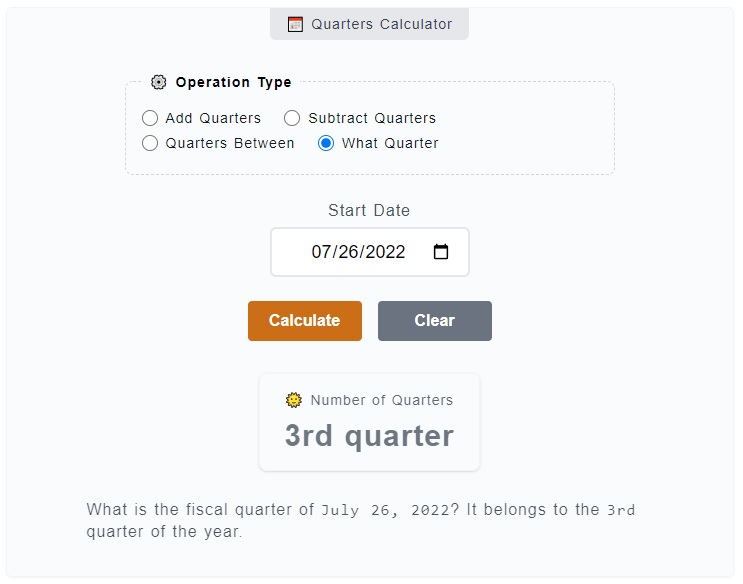# Quarters Calculator

The Quarters Calculator has four (4) functionalities, it is used to get the number of quarters between two dates, add quarters, subtract quarters and determine the fiscal quarter of a date. For example, you can add `9 quarters` or subtract `3` quarters from the starting date. You can also get the quarters between and . Just enter these values on the calculator.

📅 Quarters Calculator
⚙️ Operation Type

### 🌞 Number of Quarters

12 quarters

How many quarters are there between `June 23, 2023` and `June 23, 2026`? There is a total of `12` quarters.

## How to Use the Quarters Calculator

To use the Quarters Calculator, you just need to pick first the operation you want to use - add quarters, subtract quarters or get the total number of quarters in between two dates. You just need to enter the values on corresponding inputs and click on `Calculate` button. Here's a more detailed step by step guide.1. Step 1

On the topmost part of the calculator, you can find the `⚙️ Operation Type` field set. It is where you need to choose from the operation options - add quarters, subtract quarters and quarters between. For this example, let's select the `quarters between` option which is already selected by default.

2. Step 2

After you have chosen an operation type, the next thing to do is to fill the input fields - `Start Date` and `End Date`. The Start Date represents the base or reference date of the count-off while the End Date is where the count-off ends.

3. Step 3

The final step is to click on the `Calculate` button to get your results. You can reset the filled input fields as well by just clicking on the `Clear` button.

In case you are doing some addition or subtraction of quarters from the starting date, first select the `add` or `subtract` as the type of operation. Then, enter the starting date and the number of days want to add or subtract on their corresponding fields.